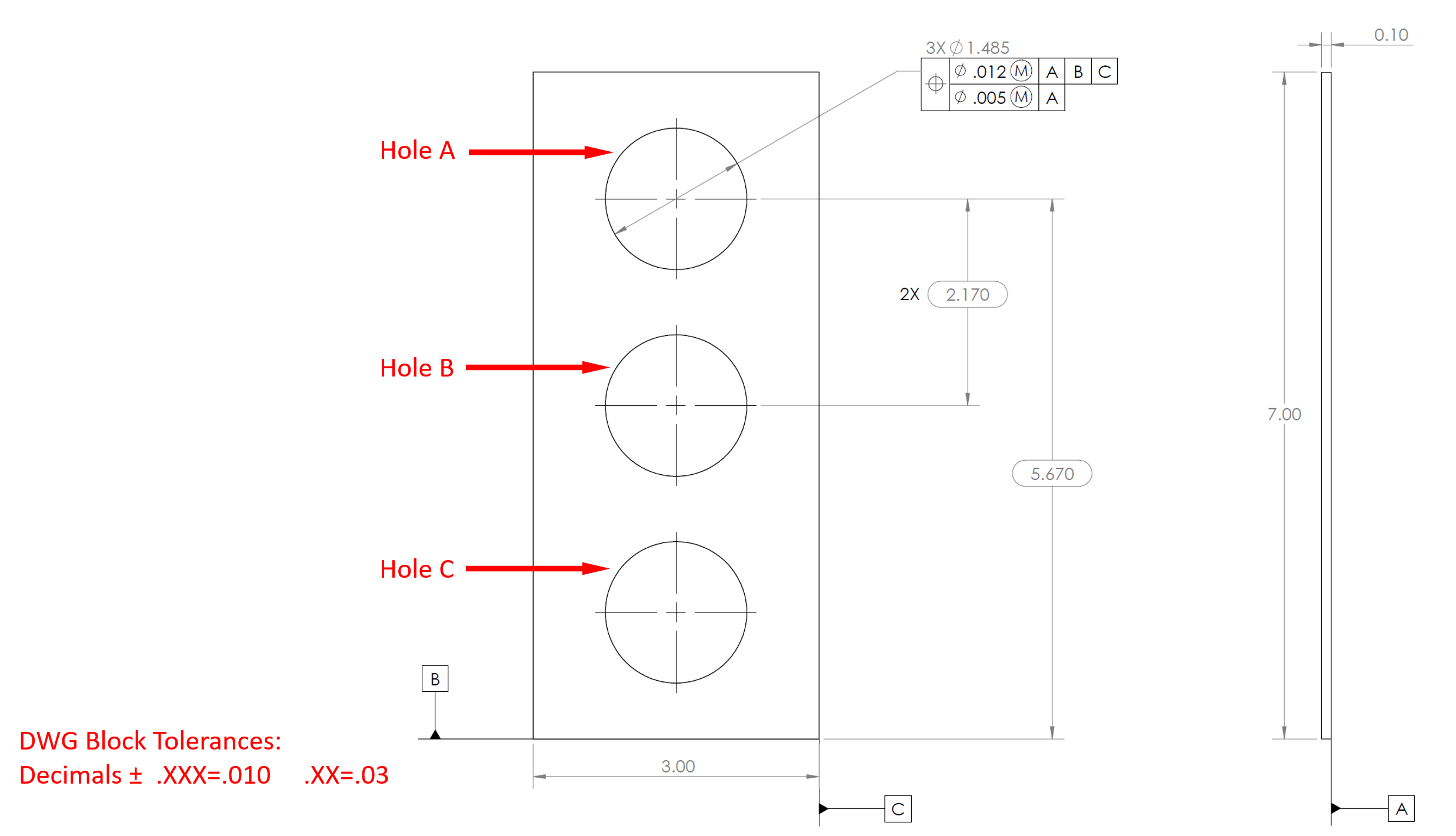## Tolerance Analysis

Consider the below drawing of a front panel plate cutout. Assume the 3 holes line up perfectly along the vertical centerline.
1. What is the largest vertical spacing allowed between Holes B and C?
2. What is the largest diameter allowed for Hole B?
3. What is the smallest distance allowed between Hole A and Datum B?Hint
All the basic dimensions are controlled by the Composite Positional Tolerance. All bilateral dimensions are controlled by the block tolerances.
Hint 2
The tolerances will determine how much you subtract/add between dimensions. The question is basically asking the min and max distances/diameter.
1. The vertical spacings between holes A-B and B-C are controlled by the "2X 2.170" dimension, and are identical. Because "2.170" is a basic dimension, the Composite Positional Tolerance associated with the Hole A diameter callout will dictate the max range. The upper segment dictates the positional tolerance of Hole A relative to Datum B, while the lower segment determines the tolerance for the spacings between all three holes.
$$2.170+(.005/2)=2.1725\:max\:distance$$$2. The "3X ⌀1.485" callout for Hole A is a bilateral dimension that controls the diameters for all three holes. As a result, the drawing block tolerance controls this feature. Because the "⌀1.485" callout has three decimal places: $$1.485+.010=1.495\:max\:diameter$$$
3. The spacing between Hole A and Datum B is controlled by the "5.670" dimension. Because "5.670" is a basic dimension, the Composite Positional Tolerance associated with the Hole A diameter callout will dictate the max distance. The upper segment dictates the positional tolerance of Hole A relative to Datum B, while the lower segment determines the tolerance for the spacings between all three holes.
$$5.670-(.012/2)=5.664\:min\:distance$$\$
1. 2.1725 max distance
2. 1.495 max distance
3. 5.664 min distance